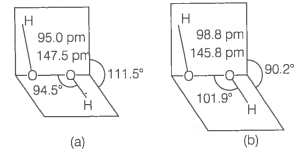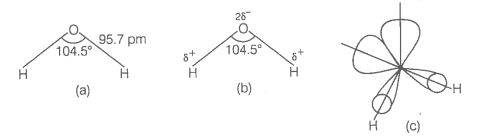How will you concentrate ${H}_{2}{O}_{2}$ ? Show differences between the structures of ${H}_{2}{O}_{2}$ and ${H}_{2}O$ by drawing the spatial structures . Also mention three uses of ${H}_{2}{O}_{2}$.

(i) Industrially, ${\mathrm{H}}_{2}{\mathrm{O}}_{2}$ is prepared by the auto-oxidation of 2-alkylanthraquinols.
In this case, 1% ${\mathrm{H}}_{2}{\mathrm{O}}_{2}$ is formed. It is extracted with water and concentrated to $~$30% (by mass) by distillation under reduced pressure.
It can be further concentrated to $~$85% by careful distillation under low pressure. The remaining water can be frozen out to obtain pure ${\mathrm{H}}_{2}{\mathrm{O}}_{2}$.
(ii) ${\mathrm{H}}_{2}{\mathrm{O}}_{2}$ has a non-planar structure.
The molecular dimensions in the gas phase and solid phase are shown below(a) ${\mathrm{H}}_{2}{\mathrm{O}}_{2}$ structure in gas phase, dihedral angle is $111.5°$
(b) ${\mathrm{H}}_{2}{\mathrm{O}}_{2}$ structure in solid phase at 110 K, dihedral angle is $90.2°$
In the gas phase, ${\mathrm{H}}_{2}\mathrm{O}$ is a bent molecule with a bond angle of $104.5°$ and O-H bond length of 95.7 pm as shown below(a) The bent structure of water;
(b) The water molecule as a dipole and
(c) The orbital overlap picture in water molecule.
(iii) Following are the three important uses of ${\mathrm{H}}_{2}{\mathrm{O}}_{2}$
(a) In daily life, it is used as a hair bleach and as a mild disinfectant. As an antiseptic it is sold in the market as perhydrol.
(b) It is used in the synthesis of hydroquinone, tartaric acid and certain food products and pharmaceuticals (cephalosporin) etc.
(c) It is employed in the industries as a bleaching agent for textiles, paper pulp, leather, oils, fats etc.